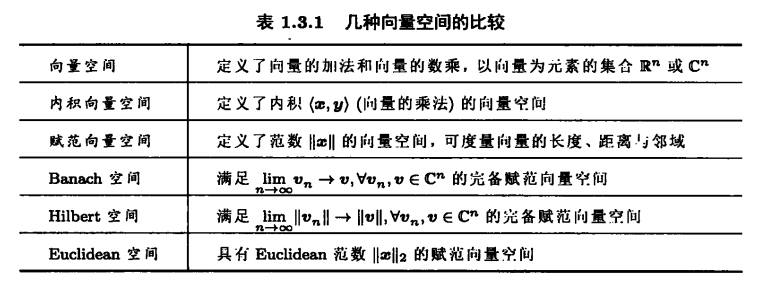# 向量空间## 1. 向量空间（线性空间）

### 1.1 定义

#### 1.1.1 向量空间

• 闭合性
1. $\boldsymbol{x},\boldsymbol{y} \in \boldsymbol{V}$，则 $\boldsymbol{x} + \boldsymbol{y} \in \boldsymbol{V}$，即 $\boldsymbol{V}$ 在加法下闭合，简称为加法的闭合性
2. $a \in \mathbb{K}$$\boldsymbol{y} \in \boldsymbol{V}$，则 $a \boldsymbol{y} \in \boldsymbol{V}$，即 $\boldsymbol{V}$ 在标量乘法下是闭合的，简称标量乘法的闭合性
• 加法的公理
1. $\boldsymbol{x} + \boldsymbol{y} = \boldsymbol{y} + \boldsymbol{x}$，称为加法交换律
2. $\boldsymbol{x} + (\boldsymbol{y} + \boldsymbol{z}) = (\boldsymbol{x} + \boldsymbol{y}) + \boldsymbol{z}$，称为加法的结合律
3. $\boldsymbol{V}$ 存在零向量 $0$，使得 $\forall \boldsymbol{y} \in \boldsymbol{V}$，恒有 $\boldsymbol{y} + \boldsymbol{0} = \boldsymbol{y}$，称为零向量的存在性
4. $\forall \boldsymbol{y} \in \boldsymbol{V}, \exists -\boldsymbol{y} \in \boldsymbol{V}$，使得 $\boldsymbol{y} + (-\boldsymbol{y}) = (-\boldsymbol{y}) + \boldsymbol{y} = \boldsymbol{0}$，称为负向量的存在性
• 标量乘法的公理
1. $a(b\boldsymbol{y}) = (ab)\boldsymbol{y}$ 对所有向量 $\boldsymbol{y}$ 和所有标量 $a,b$ 成立，称为标量乘法结合律
2. $a(\boldsymbol{x} + \boldsymbol{y}) = a\boldsymbol{x} + b\boldsymbol{y}$ 对所有向量 $\boldsymbol{y}$ 和所有标量 $a,b$ 成立，称为标量乘法分配律
3. $(a+b)\boldsymbol{y} = a\boldsymbol{y} + b\boldsymbol{y}$ 对所有向量 $\boldsymbol{y}$ 和所有标量 $a,b$ 成立，称为标量乘法分配律
4. $1\boldsymbol{y} = \boldsymbol{y}$ 对所有 $\boldsymbol{y}$ 成立，称为标量乘法单位律

#### 1.1.2 向量子空间

$\boldsymbol{V}$$\boldsymbol{W}$ 是两个向量空间，若 $\boldsymbol{W}$$\boldsymbol{V}$ 中一个非空子集合，则称子集合 $\boldsymbol{W}$$\boldsymbol{V}$ 的一个子空间。

• $\boldsymbol{A}$$\boldsymbol{B}$ 是向量空间 $\boldsymbol{V}$ 的两个向量子空间，则称

$\begin{array}{c} \boldsymbol{A} + \boldsymbol{B} = \{\boldsymbol{x} + \boldsymbol{y} | \boldsymbol{x} \in \boldsymbol{A}, \boldsymbol{y} \in \boldsymbol{B}\} \end{array}$

$\begin{array}{c} \boldsymbol{A} \cap \boldsymbol{B} = \{\boldsymbol{x} \in \boldsymbol{V} | \boldsymbol{x} \in \boldsymbol{A} \wedge \boldsymbol{x} \in \boldsymbol{B}\} \end{array}$

• $\boldsymbol{A}$$\boldsymbol{B}$ 是向量空间 $\boldsymbol{V}$ 的两个向量子空间，并满足

$\begin{array}{c} \boldsymbol{V} = \boldsymbol{A} + \boldsymbol{B} \\ \boldsymbol{A} \cap \boldsymbol{B} = \{\boldsymbol{0}\} \end{array}$

#### 1.1.3 线性映射（线性变换）

$\boldsymbol{V}$$\boldsymbol{W}$ 分别是 $\mathbb{R}^m$$\mathbb{R}^n$ 的子空间，若映射 $\boldsymbol{T}: \boldsymbol{V} \mapsto \boldsymbol{W}$$\boldsymbol{v} \in \boldsymbol{V}, \boldsymbol{w} \in \boldsymbol{W}$ 和任意标量 $c$ 满足叠加性齐次性，则称 $\boldsymbol{T}$线性映射线性变换

• 叠加性：$\boldsymbol{T}(\boldsymbol{v} + \boldsymbol{w}) = \boldsymbol{T}(\boldsymbol{v}) + \boldsymbol{T}(\boldsymbol{w})$

• 齐次性：$\boldsymbol{T}(c\boldsymbol{v}) = c\boldsymbol{T}(\boldsymbol{v})$

$\begin{array}{c} \boldsymbol{T}(c_1 \boldsymbol{v} + c_2 \boldsymbol{w}) = c_1 \boldsymbol{T}(\boldsymbol{v}) + c_2 \boldsymbol{T}(\boldsymbol{w}) \end{array}$

### 1.2 性质

• 常见向量空间：$\mathbb{R}^n, \mathbb{C}^n$

• $\mathbb{R}^n$ 的子集合 $\boldsymbol{W}$$\mathbb{R}^n$ 的一个子空间，当且仅当以下三个条件满足：

1. $\boldsymbol{x},\boldsymbol{y} \in \boldsymbol{W}$，则 $\boldsymbol{x} + \boldsymbol{y} \in \boldsymbol{W}$，即满足加法的闭合性。
2. $a \in \boldsymbol{S}$$\boldsymbol{y} \in \boldsymbol{W}$，则 $a \boldsymbol{y} \in \boldsymbol{W}$，即满足标量乘法的闭合性。
3. 满足零向量的存在性。

## 2. 内积空间

### 2.1 定义

#### 2.1.1 内积空间

$\boldsymbol{V}$ 为向量空间，若对所有 $\boldsymbol{x},\boldsymbol{y},\boldsymbol{z} \in \boldsymbol{V}$$a,b \in \mathbb{K}$，映射函数 $\langle \cdot, \cdot \rangle: \boldsymbol{V} \times \boldsymbol{V} \mapsto \mathbb{K}$ 满足以下三条性质：

• 共轭对称性：$\langle \boldsymbol{x}, \boldsymbol{y} \rangle = \langle \boldsymbol{y}, \boldsymbol{x} \rangle^*$

• 第一变元的线性性：$\langle a\boldsymbol{x} + b\boldsymbol{y}, \boldsymbol{z} \rangle = a \langle \boldsymbol{x}, \boldsymbol{z} \rangle + b \langle \boldsymbol{y}, \boldsymbol{z} \rangle$

• 非负性：$\langle \boldsymbol{x}, \boldsymbol{x} \rangle \geq 0$，且 $\langle \boldsymbol{x}, \boldsymbol{x} \rangle = 0 \Leftrightarrow \boldsymbol{x} = \boldsymbol{0}$

### 2.2 性质

• $\forall \mathbf{x} \in \mathbf{V}, \langle \mathbf{x},\mathbf{x} \rangle = \langle \mathbf{x},\mathbf{x} \rangle^* \in \mathbb{R}$。（共轭对称性）
• $\forall a,b \in \mathbb{K}, \mathbf{x},\mathbf{y},\mathbf{z} \in \mathbf{V}, \langle \mathbf{z}, a\mathbf{x}+b\mathbf{y} \rangle = a^* \langle \mathbf{z},\mathbf{x} \rangle + b^* \langle \mathbf{z},\mathbf{y} \rangle$。（第一变元的线性性）

## 3. 赋范空间

### 3.1 定义

#### 3.1.1 赋范空间

$\boldsymbol{V}$ 为向量空间，向量 $\boldsymbol{x}$ 的范数为一实函数 $p(\boldsymbol{x}): \boldsymbol{V} \mapsto \mathbb{R}$，若对所有向量 $\boldsymbol{x},\boldsymbol{y} \in \boldsymbol{V}$ 和任意一个标量 $c \in \mathbb{K}$，有下面性质成立：

• 非负性：$p(\boldsymbol{x}) \geq 0$$p(\boldsymbol{x}) = 0 \Leftrightarrow \boldsymbol{x} = \boldsymbol{0}$

• 齐次性：$p(c\boldsymbol{x}) = |c| \cdot p(\boldsymbol{x})$ 对任意标量 $c$ 成立。

• 三角不等式：$p(\boldsymbol{x} + \boldsymbol{y}) \leq p(\boldsymbol{x}) + p(\boldsymbol{y})$

#### 3.1.2 半范数（伪范数）

$p(\boldsymbol{x}): \boldsymbol{V} \mapsto \mathbb{R}$ 对所有向量 $\boldsymbol{x}, \boldsymbol{y} \in \boldsymbol{V}$ 和任意一个标量 $c$，有以下条件成立：

• $p(\boldsymbol{x}) \geq 0$

• $p(c\boldsymbol{x}) = |c| \cdot p(\boldsymbol{x})$

• $p(\boldsymbol{x}+\boldsymbol{y}) \leq p(\boldsymbol{x}) + p(\boldsymbol{y})$

【注】半范数与范数的唯一区别在于：半范数不完全满足范数的非负性条件。

#### 3.1.3 拟范数

$p(\boldsymbol{x}): \boldsymbol{V} \mapsto \mathbb{R}$ 对所有向量 $\boldsymbol{x}, \boldsymbol{y} \in \boldsymbol{V}$ 和任意一个标量 $c$，有以下条件成立：

• $p(\boldsymbol{x}) \geq 0$$p(\boldsymbol{x}) = 0 \Leftrightarrow \boldsymbol{x} = \boldsymbol{0}$

• $p(c\boldsymbol{x}) = |c| \cdot p(\boldsymbol{x})$

• $p(\boldsymbol{x}+\boldsymbol{y}) \leq C(p(\boldsymbol{x}) + p(\boldsymbol{y}))$，其中 $C \in \mathbb{R}, C \gt 0 \wedge C \ne 1$

【注】拟范数和范数的唯一区别在于：拟范数不满足范数的三角不等式。

### 3.1.4 完备性

• $\boldsymbol{V}$ 为向量空间，若对于 $\boldsymbol{V}$ 中的每一个 Cauchy 序列 $\{\boldsymbol{v}_n\}_{n=1}^{\infty} \subset \boldsymbol{V}$，在向量空间 $\boldsymbol{V}$ 内都存在一个向量 $\boldsymbol{v}$，使得 $\lim_{n \rightarrow \infty} \boldsymbol{v}_n \rightarrow \boldsymbol{v}$，即 $\boldsymbol{V}$ 中每一个 Cauchy 序列都收敛在向量空间 $\boldsymbol{V}$ 内，则称向量空间 $\boldsymbol{V}$完备向量空间
• 类似地，令 $\boldsymbol{V}$ 为赋范向量空间，若对于 $\boldsymbol{V}$ 中的每一个 Cauchy 序列 $\{\boldsymbol{v}_n\}_{n=1}^{\infty} \subset \boldsymbol{V}$，在赋范向量空间 $\boldsymbol{V}$ 内都存在一个向量 $\boldsymbol{v}$，使得 $\lim_{n \rightarrow \infty} \parallel\boldsymbol{v}_n\parallel \rightarrow \parallel\boldsymbol{v}\parallel$，即 $\boldsymbol{V}$ 中每一个 Cauchy 序列都收敛在赋范向量空间 $\boldsymbol{V}$ 内，则称赋范向量空间 $\boldsymbol{V}$完备赋范向量空间

## 4. Banach 空间

### 4.1 定义

$\boldsymbol{V}$ 为赋范向量空间，若对每一个 Cauchy 序列 $\{\boldsymbol{v}_n\}_{n=1}^{\infty} \subset \boldsymbol{V}$，在 $\boldsymbol{V}$ 都存在一个向量 $\boldsymbol{v}$，使得 $\lim_{n \rightarrow \infty} \boldsymbol{v}_n \rightarrow \boldsymbol{v}$，则称 $\boldsymbol{V}$Banach 空间